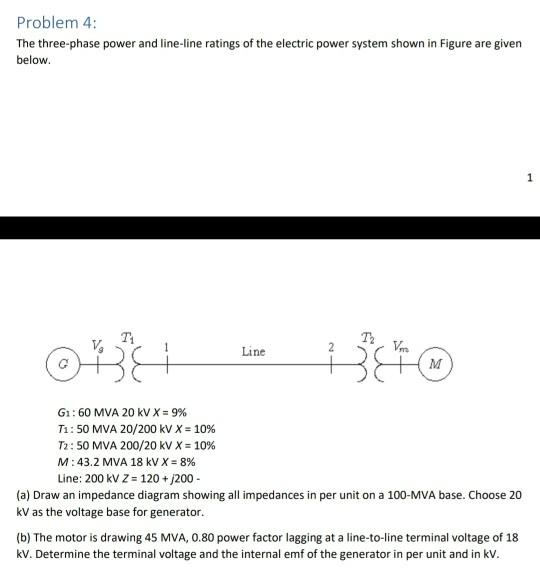# Question Problem 4: The three-phase power and line-line ratings of the electric power system shown in Figure are given below. 1 T! Line M 61: 60 MVA 20 KV X = 9% T1: 50 MVA 20/200 kV X = 10% T2: 50 MVA 200/20 kV X = 10% M:43.2 MVA 18 kV X = 8% Line: 200 KV Z = 120 + 200 - (a) Draw an impedance diagram showing all impedances in per unit on a 100-MVA base. Choose 20 kV as the voltage base for generator. (b) The motor is drawing 45 MVA, 0.80 power factor lagging at a line-to-line terminal voltage of 18 kv. Determine the terminal voltage and the internal emf of the generator in per unit and in kv.FULGCJ The Asker · Electrical EngineeringTranscribed Image Text: Problem 4: The three-phase power and line-line ratings of the electric power system shown in Figure are given below. 1 T! Line M 61: 60 MVA 20 KV X = 9% T1: 50 MVA 20/200 kV X = 10% T2: 50 MVA 200/20 kV X = 10% M:43.2 MVA 18 kV X = 8% Line: 200 KV Z = 120 + 200 - (a) Draw an impedance diagram showing all impedances in per unit on a 100-MVA base. Choose 20 kV as the voltage base for generator. (b) The motor is drawing 45 MVA, 0.80 power factor lagging at a line-to-line terminal voltage of 18 kv. Determine the terminal voltage and the internal emf of the generator in per unit and in kv.
More
Transcribed Image Text: Problem 4: The three-phase power and line-line ratings of the electric power system shown in Figure are given below. 1 T! Line M 61: 60 MVA 20 KV X = 9% T1: 50 MVA 20/200 kV X = 10% T2: 50 MVA 200/20 kV X = 10% M:43.2 MVA 18 kV X = 8% Line: 200 KV Z = 120 + 200 - (a) Draw an impedance diagram showing all impedances in per unit on a 100-MVA base. Choose 20 kV as the voltage base for generator. (b) The motor is drawing 45 MVA, 0.80 power factor lagging at a line-to-line terminal voltage of 18 kv. Determine the terminal voltage and the internal emf of the generator in per unit and in kv.